# Comparing Temperatures

Comparing Temperatures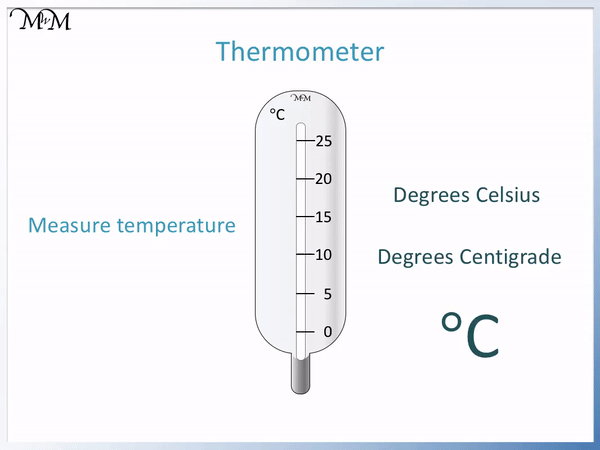• A thermometer is used to measure temperature, which is how hot something is.
• There is a liquid called mercury inside a thermometer which rises up the thermometer as it gets warmer.
• The temperature reading is the number on the scale of the thermometer that the mercury rises to be level with.
• We measure temperature with degrees Celsius (which used to be called centigrade).
• Degrees Celsius is written as °C for short.
• This thermometer scale goes up in 5 °C increments, so we count up in fives for every line.

The temperature on a thermometer is the number which the liquid mercury is in line with.

We read temperature in degrees Celsius.• The temperature outside is 10 °C because the mercury is in line with the 10 on the thermometer scale.
• The temperature inside the house is 18 °C.
• It is warmer inside the house because 18 is larger than 10
• To find out how much warmer we can count on from 10 to get to 18.
• We have: 11, 12 , 13, 14, 15, 16, 17 and 18. This is 8 more numbers, so the temperature is 8 °C warmer than outside.
• In maths, difference means subtract and so we can find the temperature difference by subtracting the lower temperature from the larger temperature.
• 18 – 10 = 8 and so the temperature is 8 °C warmer inside.
• We can write 18 °C > 10 °C to say that 18 is greater than 10.# How to Compare Temperatures using a Thermometer

Temperature is a measure of how hot something is. The larger the temperature, the hotter it is. The lower the temperature, the cooler it is.

To measure temperature we use a plastic rod called a thermometer. A thermometer contains a liquid called mercury. The mercury expands as it gets warmer and the hotter it is, the more the mercury rises upwards through the thermometer tube.

There are numbers on the side of the thermometer scale which help us to record the temperature. The temperature on a thermometer is the number which the mercury is in line with.

Here is an animation showing the temperature on a thermometer rising as it gets warmer.Temperature is measure in degrees Celsius.

We can write degrees with the symbol ‘°’ for short.

We write Celsius with a ‘C’ for short.

So to write temperature in degrees Celsius we write °C after the number.On the thermometer shown in the image above, the numbers on the scale go up in fives.

The mercury is in line with the number 15, which means that the temperature reading is 15 °C.

To compare temperatures we read the temperature and the the larger reading will be the warmer temperature.

To calculate the difference between two temperatures subtract the smaller temperature from the larger temperature.

In this example, we will compare the temperatures on two different days.

The thermometer is in line with 5 °C on Monday.

The thermometer is in line with 10 °C on Tuesday.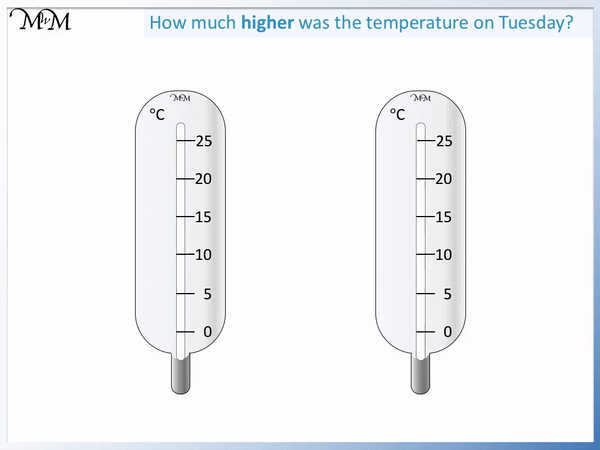To find the difference in temperature between the two days, we subtract the smaller temperature from the larger temperature.

10 – 5 = 5

The difference in temperature is 5 °C.

It is 5 °C warmer on Tuesday than it is on Monday.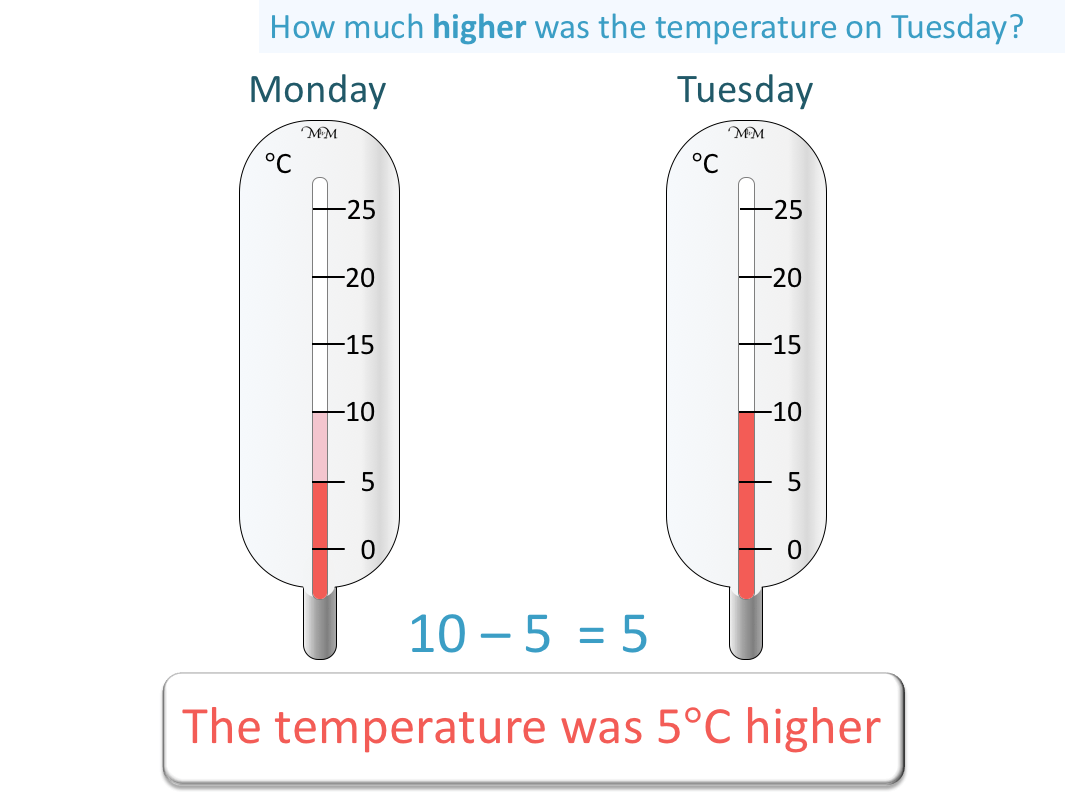We can also see that the thermometer is one increment higher on the scale on Tuesday compared to Monday.

Each increment is worth 5 °C on this thermometer.

In this next example we will compare the temperatures inside and outside a house.

This time, the increments on the thermometer are going up in twos. The numbers on the side of the thermometer go up in twos.

The thermometer is in line with 8 °C outside.

The thermometer is in line with 18 °C inside.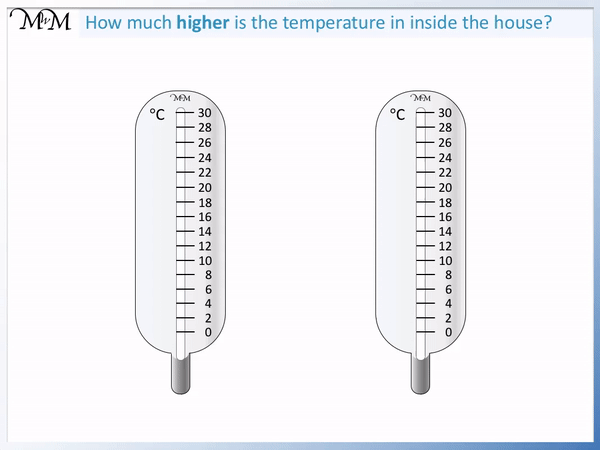Because the temperature is a larger number inside compared to outside, we say it is warmer inside compared to outside.

The difference in temperature is found by subtracting the smaller number from the larger number.

18 – 8 = 10

And so, the difference in temperature is 8 °C.

We can say that it is 8 °C warmer inside compared to outside.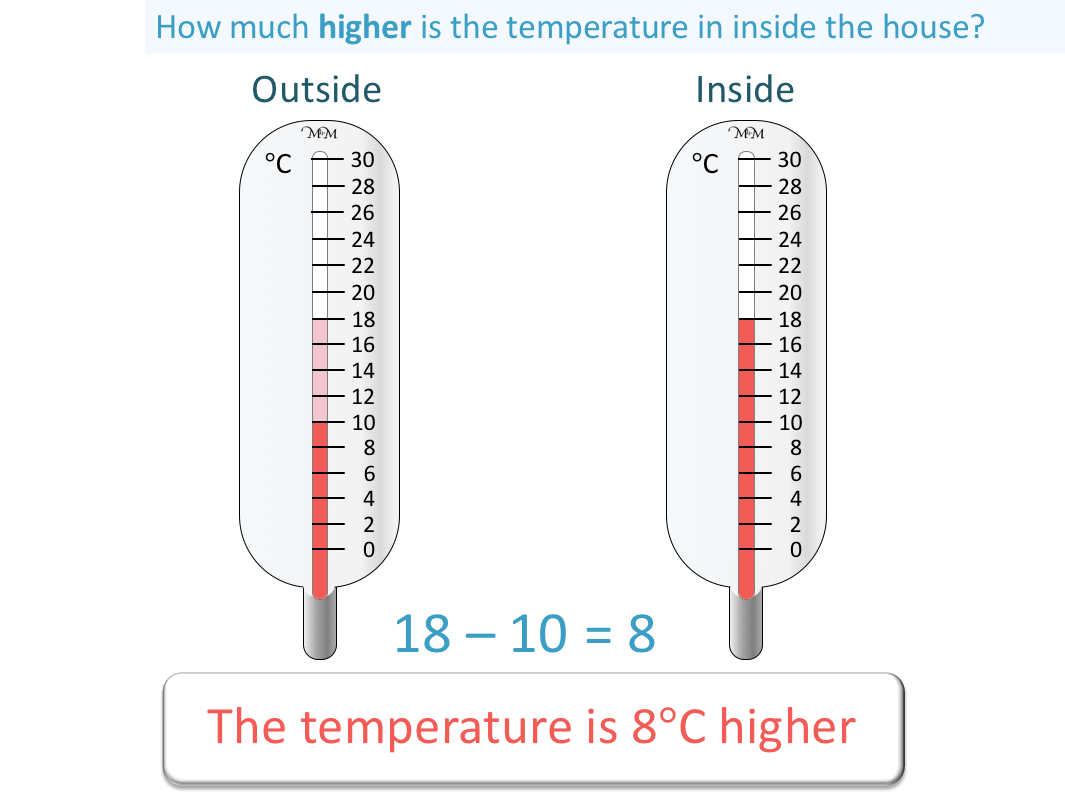We can see that the mercury is higher up the thermometer when it is warmer.

To compare the temperature easily, we can just look to see on which thermometer the mercury is the highest.

In this example we will compare the temperatures in two cities.

Again the scale on the thermometer is going up in twos.

The thermometer is in line with 26 °C in Sydney.

The thermometer is directly between 22 °C and 24 °C in London.

The number directly half way between 22 and 24 is 23.

The temperature in London is 23 °C.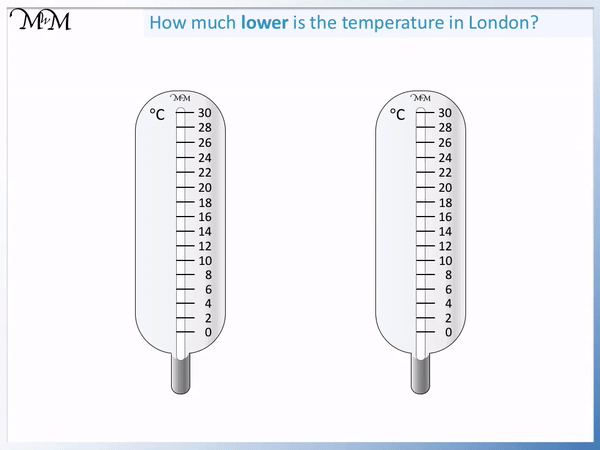26 °C is larger than 23 °C and so, it is warmer in Sydney than in London.

The mercury on the thermometer is higher in Sydney compared to London.

The difference in temperature is found by subtracting the lower temperature from the larger temperature.

26 – 23 = 3And so, the temperature in Sydney is 3 °C higher than in London.

We can also write that 26 °C > 23 °C to say that ’26 °C is greater than 23 °C’.

The ‘>’ sign reads as ‘greater than’.

The ‘>’ sign reads as ‘less than’.

The open end of the sign always opens out to face the larger number and the closed tip of the sign points at the smaller number.

In this next example we will compare temperatures at night and day.

The thermometer is in the daytime is 2 °C above 15 °C.

15 + 2 = 17

The thermometer in the daytime is therefore at 17 °C.

The thermometer in the nighttime is 3 °C above 5 °C.

5 + 3 = 8

The nighttime temperature is therefore at 8 °C.

17 °C is larger than 8 °C and so, it is warmer in the daytime than at nighttime.

We can write 17 °C > 8 °C. The ‘arrow’ of this sign points at the smaller temperature.The difference in temperature is 17 – 8, which equals 9.

We can say that it is 9 °C warmer in the day than at night.Now try our lesson on Measuring Centimetres Using a Ruler where we learn how to measure centimetres using a ruler.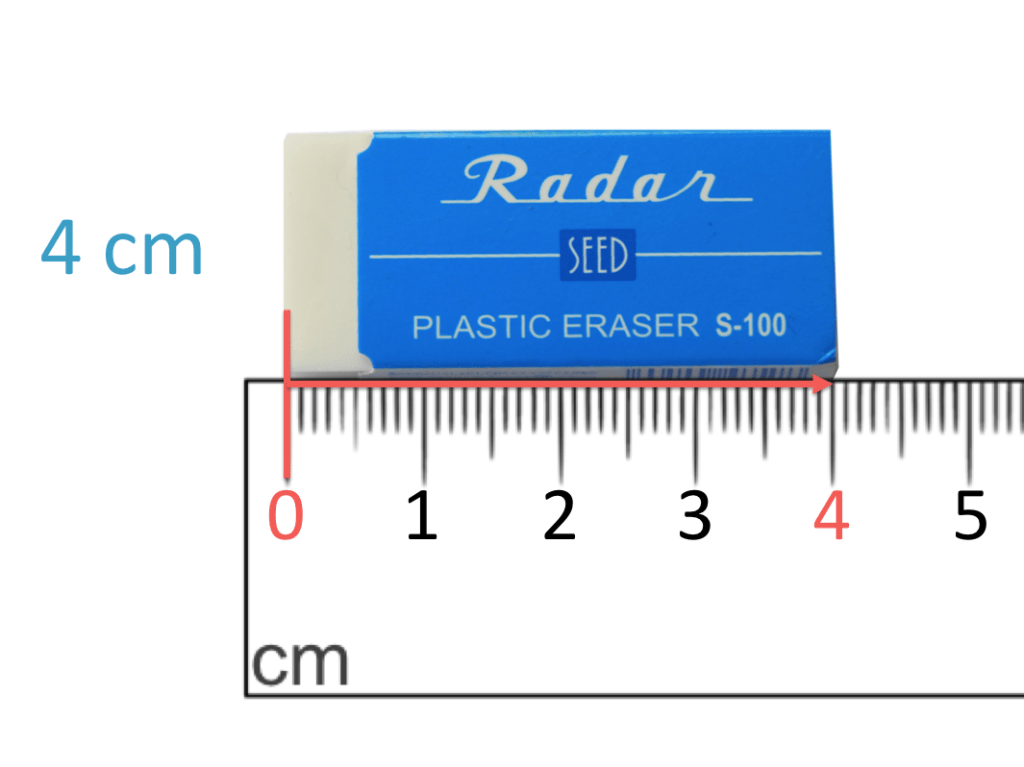error: Content is protected !!